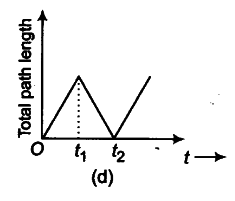# Look at the graphs (a) to (d) carefully and state

Look at the graphs (a) to (d) carefully and state with reasons, which of these cannot possibly represent one-dimensional motion of a particle?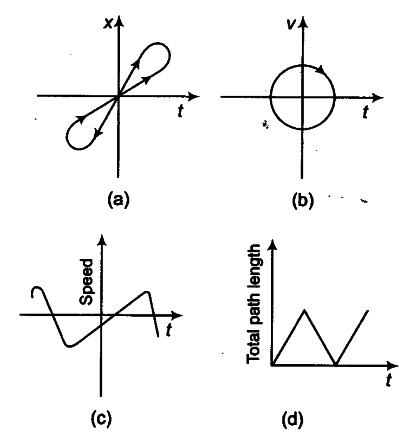(a) No, graph (a) is not representing one-dimensional motion of a particle, because graph shows two different positions of the particle at same instant of time. (At time { t }_{ 1 }, particle is at positions P and Q and at time
{ t }_{ 2 }, particle is at positions R and S), which is not possible.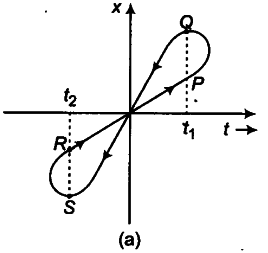(b) No, graph (b) cannot represent one-dimensional motion of a particle, because graph shows one positive velocity ({ v }_{ 1 }) and another negative velocity
(-{ v }_{ 2 }) of the particle at the same instant of time {
{ t }_{ 1 }) which is not possible.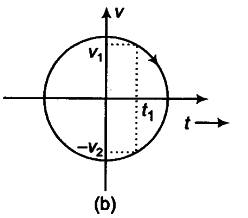© No, graph © cannot represent one-dimensional motion of a particle, because graph shows negative speed of the particle but speed cannot be negative.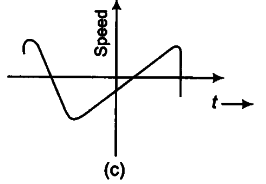{d) No, graph (d) cannot represent one-dimensional motion of a particle, because graph shows that total path length increases from time t = 0 to t ={ t }_{ 1 }, but decreases from t =
{ t }_{ 1 } to t =
{ t }_{ 2 }. But total path length of a moving particle can never decrease with time.